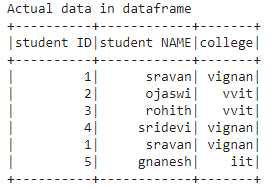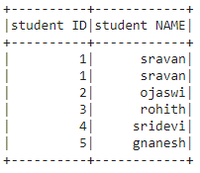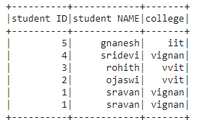# How to select and order multiple columns in Pyspark DataFrame ?

• Last Updated : 06 Jun, 2021

In this article, we will discuss how to select and order multiple columns from a dataframe using pyspark in Python. For this, we are using sort() and orderBy() functions along with select() function.

### Methods Used

• Select(): This method is used to select the part of dataframe columns and return a copy of that newly selected dataframe.

Syntax: dataframe.select([‘column1′,’column2′,’column n’].show()

• sort(): This method is used to sort the data of the dataframe and return a copy of that newly sorted dataframe. This sorts the dataframe in ascending by default.

Syntax: dataframe.sort([‘column1′,’column2′,’column n’], ascending=True).show()

• oderBy(): This method is similar to sort which is also used to sort the dataframe.This sorts the dataframe in ascending by default.

Syntax: dataframe.orderBy([‘column1′,’column2′,’column n’], ascending=True).show()

Let’s create a sample dataframe

## Python3

 `# importing module``import` `pyspark`` ` `# importing sparksession from ``# pyspark.sql module``from` `pyspark.sql ``import` `SparkSession`` ` `# creating sparksession and giving an app name``spark ``=` `SparkSession.builder.appName(``'sparkdf'``).getOrCreate()`` ` `# list  of students  data``data ``=` `[[``"1"``, ``"sravan"``, ``"vignan"``], [``"2"``, ``"ojaswi"``, ``"vvit"``],``        ``[``"3"``, ``"rohith"``, ``"vvit"``], [``"4"``, ``"sridevi"``, ``"vignan"``],``        ``[``"1"``, ``"sravan"``, ``"vignan"``], [``"5"``, ``"gnanesh"``, ``"iit"``]]`` ` `# specify column names``columns ``=` `[``'student ID'``, ``'student NAME'``, ``'college'``]`` ` `# creating a dataframe from the lists of data``dataframe ``=` `spark.createDataFrame(data, columns)`` ` `print``(``"Actual data in dataframe"``)``# show dataframe``dataframe.show()`

Output:Selecting multiple columns and order by using sort() method

## Python3

 `# show dataframe by sorting the dataframe``# based on two columns in ascending``# order using sort() function``dataframe.select([``'student ID'``, ``'student NAME'``]``                ``).sort([``'student ID'``, ``'student NAME'``], ``                       ``ascending``=``True``).show()`

Output:## Python3

 `# show dataframe by sorting the dataframe``# based on three columns in desc order``# using sort() function``dataframe.select([``'student ID'``, ``'student NAME'``, ``'college'``]``                ``).sort([``'student ID'``, ``'student NAME'``, ``'college'``],``                       ``ascending``=``False``).show()`

Output:Selecting multiple columns and order by using orderBy() method

## Python3

 `# show dataframe by sorting the dataframe``# based on three columns in desc``# order using orderBy() function``dataframe.select([``'student ID'``, ``'student NAME'``, ``'college'``]``                ``).orderBy([``'student ID'``, ``'student NAME'``, ``'college'``],``                          ``ascending``=``False``).show()`

Output:## Python3

 `# show dataframe by sorting the dataframe``# based on two columns in asc``# order using orderBy() function``dataframe.select([``'student NAME'``, ``'college'``]``                ``).orderBy([``'student NAME'``, ``'college'``],``                          ``ascending``=``True``).show()`

Output:My Personal Notes arrow_drop_up